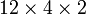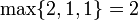# Direct product of A4 and Z4 and Z2

Jump to: navigation, search
This article is about a particular group, i.e., a group unique upto isomorphism. View specific information (such as linear representation theory, subgroup structure) about this group
View a complete list of particular groups (this is a very huge list!)[SHOW MORE]

## Definition

This group is defined in the following equivalent ways:

1. It is the external direct product of alternating group:A4, cyclic group:Z4, and cyclic group:Z2.
2. It is the external direct product of alternating group:A4 and direct product of Z4 and Z2.
3. It is the external direct product of cyclic group:Z4 and direct product of A4 and Z2.
4. It is the external direct product of cyclic group:Z2 and direct product of A4 and Z4.

## Arithmetic functions

Want to compare and contrast arithmetic function values with other groups of the same order? Check out groups of order 96#Arithmetic functions
Function Value Similar groups Explanation
order (number of elements, equivalently, cardinality or size of underlying set) 96 groups with same order order of direct product is product of orders: the order is$12 \times 4 \times 2$, where$12 = 4!/2$ is the order of alternating group:A4, 4 is the order of cyclic group:Z4 and 2 is the order of cyclic group:Z2.
exponent of a group 12 groups with same order and exponent of a group | groups with same exponent of a group exponent of direct product is lcm of exponents: the exponent is$\operatorname{lcm}\{6,4,2 \} = 12$.
minimum size of generating set 2 groups with same order and minimum size of generating set | groups with same minimum size of generating set
derived length 2 groups with same order and derived length | groups with same derived length derived length of direct product is maximum of derived lengths: the derived length is thus$\operatorname{max} \{ 2,1,1 \} = 2$.

## GAP implementation

### Group ID

This finite group has order 96 and has ID 196 among the groups of order 96 in GAP's SmallGroup library. For context, there are 231 groups of order 96. It can thus be defined using GAP's SmallGroup function as:

SmallGroup(96,196)

For instance, we can use the following assignment in GAP to create the group and name it$G$:

gap> G := SmallGroup(96,196);

Conversely, to check whether a given group$G$ is in fact the group we want, we can use GAP's IdGroup function:

IdGroup(G) = [96,196]

or just do:

IdGroup(G)

to have GAP output the group ID, that we can then compare to what we want.

### Other descriptions

Description Functions used
DirectProduct(AlternatingGroup(4),CyclicGroup(4),CyclicGroup(2)) DirectProduct, AlternatingGroup, CyclicGroup